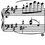# Armstrong number

I just came across the post on the Facebook page Art of Mathematics, which says:

$$\textcolor{green}{1}^3 + \textcolor{orange}{5}^3 + \textcolor{blue}{3}^3 = \textcolor{green}{1}\textcolor{orange}{5}\textcolor{blue}{3}$$

$\textcolor{#20A900}{1}\textcolor{#D61F06}{6}^3 + \textcolor{#EC7300}{5}0^3+ \textcolor{#3D99F6}{33}^3=\textcolor{#20A900}{1}\textcolor{#D61F06}{6}\textcolor{#EC7300}{5}0\textcolor{#3D99F6}{33}$

$\textcolor{#20A900}{1}\textcolor{#D61F06}{66}^3 + \textcolor{#EC7300}{5}00^3+ \textcolor{#3D99F6}{333}^3=\textcolor{#20A900}{1}\textcolor{#D61F06}{66}\textcolor{#EC7300}{5}00\textcolor{#3D99F6}{333}$

$\textcolor{#20A900}{1}\textcolor{#D61F06}{666}^3 + \textcolor{#EC7300}{5}000^3+ \textcolor{#3D99F6}{3333}^3=\textcolor{#20A900}{1}\textcolor{#D61F06}{666}\textcolor{#EC7300}{5}000\textcolor{#3D99F6}{3333}$

and so on.

And here is the proof:

Let $A=\underbrace{1...6}_{n\ 6's}^{n} +\underbrace{5...0}_{n\ 0's}^{n} +\underbrace{3...3}_{n+1 \ 3's}^{n}$

We have:

$1...6^{3} \ =\left[ 10^{n} \ +\dfrac{2}{3}\left( 10^{n} -1\right)\right]^{3}\\ 5...0^{3} =5^{3} \times 10^{3n} =125\times 10^{3n}\\ 3...3^{3} =\left(\dfrac{10^{n+1} -1}{3}\right)^{3}$

Then

$A=\left[ 10^{n} \ +\dfrac{2}{3}\left( 10^{n} -1\right)\right]^{3} +125\times 10^{3n} +\left(\dfrac{10^{n+1} -1}{3}\right)^{3}\\ =\dfrac{500}{3} 10^{3n} -\dfrac{50}{3} 10^{2} n+\dfrac{10}{3} 10^{n} -\dfrac{1}{3}$

By multiplying $\dfrac {500} {3}$ with $10^{3n}$ using decimal places, we get:

$\dfrac{500}{3} 10^{3n} =166.\overline{6} \times 10^{3n} =166\ \underbrace{6...6}_{n\ 6's}\underbrace{6...6}_{n\ 6's}\underbrace{6...6}_{n\ 6's} .\overline{6}\$/extract_itex] Likewise, $\dfrac{50}{3} 10^{2n} =16.\overline{6} \times 10^{2n} =16\underbrace{6...6}_{n\ 6's}\underbrace{6...6}_{n\ 6's} .\overline{6}\\$ $\Rightarrow \dfrac{500}{3} 10^{3n} -\dfrac{50}{3} 10^{2n} =1\underbrace{6...6}_{n\ 6's} 5\underbrace{0...0}_{n\ 0's}\underbrace{0...0}_{n\ 0's}\\$ For the last term of the polynomial: $\dfrac{10}{3} 10^{n} -\dfrac{1}{3} =3.\overline{3} \times 10^{n} -0.\overline{3} =\underbrace{3...3}_{n+1\ 3's}\\$ Therefore, $A=\dfrac{500}{3} 10^{3n} -\dfrac{50}{3} 10^{2n} +\dfrac{10}{3} 10^{n} -\dfrac{1}{3} =1\underbrace{6...6}_{n\ 6's} 5\underbrace{0...0}_{n\ 0's}\underbrace{3...3}_{n+1\ 3's} \blacksquare$Note by Tín Phạm Nguyễn 2 years, 8 months ago This discussion board is a place to discuss our Daily Challenges and the math and science related to those challenges. Explanations are more than just a solution — they should explain the steps and thinking strategies that you used to obtain the solution. Comments should further the discussion of math and science. When posting on Brilliant: • Use the emojis to react to an explanation, whether you're congratulating a job well done , or just really confused . • Ask specific questions about the challenge or the steps in somebody's explanation. Well-posed questions can add a lot to the discussion, but posting "I don't understand!" doesn't help anyone. • Try to contribute something new to the discussion, whether it is an extension, generalization or other idea related to the challenge. • Stay on topic — we're all here to learn more about math and science, not to hear about your favorite get-rich-quick scheme or current world events. MarkdownAppears as *italics* or _italics_ italics **bold** or __bold__ bold - bulleted- list • bulleted • list 1. numbered2. list 1. numbered 2. list Note: you must add a full line of space before and after lists for them to show up correctly paragraph 1paragraph 2 paragraph 1 paragraph 2 [example link](https://brilliant.org)example link > This is a quote This is a quote  # I indented these lines # 4 spaces, and now they show # up as a code block. print "hello world" # I indented these lines # 4 spaces, and now they show # up as a code block. print "hello world" MathAppears as Remember to wrap math in $$ ... $$ or \[ ... $ to ensure proper formatting.
2 \times 3 $2 \times 3$
2^{34} $2^{34}$
a_{i-1} $a_{i-1}$
\frac{2}{3} $\frac{2}{3}$
\sqrt{2} $\sqrt{2}$
\sum_{i=1}^3 $\sum_{i=1}^3$
\sin \theta $\sin \theta$
\boxed{123} $\boxed{123}$

## Comments

Sort by:

Top Newest

This is very interesting

- 2 years, 8 months ago

Log in to reply

Where does the name "Armstrong Number" come from?

- 2 years, 8 months ago

Log in to reply

I think the word "Armstrong" means repeated.

- 2 years, 8 months ago

Log in to reply

An Armstrong number is an integer of $n$ digits; the sum of its digits each one raised to the power of $n$ equals the integer itself.

For example, $153$ is an Armstrong number because $153 = 1^3 + 5^3 + 3^3$

- 2 years, 8 months ago

Log in to reply

×

Problem Loading...

Note Loading...

Set Loading...